• 抽样函数的傅里叶变换
千次阅读
2021-07-09 21:23:57

### 6.抽样信号的傅里叶变换

1.时域采样

先直接给出结论：

​ 信号 f ( t ) f(t) p ( t ) p(t) 时域抽样后得到 f s ( t ) f_s(t) f s ( t ) f_s(t) 频谱 F s ( ω ) F_s(\omega) 是连续信号 f ( t ) f(t) 频谱 F ( ω ) F(\omega) 的形状以抽样频率 ω s \omega_s 为间隔的周期性重复而得到，重复过程中，幅度被 p ( t ) p(t) 所加权。 F ( ω ) F(\omega) 在重复过程中形状不会发生变化。

（1）冲激采样

​ 求冲激采样有两种方法

第一种方法：

​ 先推导时域采样的公式，得到：
F s ( ω ) = ∑ n = − ∞ ∞ P n F ( ω − n ω s ) F_s(\omega)=\sum_{n=-\infty}^{\infty}P_nF(\omega-n\omega_s)
​ 得到冲激采样的表达式：
F s ( w ) = 1 T s ∑ n = − ∞ ∞ F ( ω − n ω s ) F_s(w)=\frac{1}{T_s}\sum_{n=-\infty}^{\infty}F(\omega-n\omega_s)
第二种方法：

利用卷积定理以及冲激采样函数的傅里叶变换
f s ( t ) = δ T ( t ) f ( t ) f_s(t)=\delta_T(t)f(t)
则有
F s ( ω ) = 1 2 π δ ω s ( ω ) ∗ F ( ω ) F_s(\omega)=\frac{1}{2\pi}\delta_{\omega_s}(\omega)*F(\omega)
得到冲激采样表达式：
F s ( w ) = 1 T s ∑ n = − ∞ ∞ F ( ω − n ω s ) F_s(w)=\frac{1}{T_s}\sum_{n=-\infty}^{\infty}F(\omega-n\omega_s)
（2）矩形脉冲抽样

同样通过两种方法计算：

第一种方法：
F s ( ω ) = E τ T s ∑ n = − ∞ ∞ S a ( n ω s τ 2 ) F ( ω − n ω s ) F_s(\omega)=\frac{E\tau}{T_s}\sum_{n=-\infty}^{\infty}Sa(\frac{n\omega_s\tau}{2})F(\omega-n\omega_s)
第二种方法：
F s ( ω ) = 1 2 π E τ ω s ∑ n = − ∞ ∞ S a ( n ω 1 τ 2 ) δ ( ω − n ω s ) ∗ F ( ω ) = E τ T s ∑ n = − ∞ ∞ S a ( n ω s τ 2 ) F ( ω − n ω s ) \begin{aligned} F_s(\omega)&=\frac{1}{2\pi}E\tau\omega_s\sum_{n=-\infty}^{\infty}Sa(\frac{n\omega_1\tau}{2})\delta(\omega-n\omega_s)*F(\omega)\\ &=\frac{E\tau}{T_s}\sum_{n=-\infty}^{\infty}Sa(\frac{n\omega_s\tau}{2})F(\omega-n\omega_s) \end{aligned}
结论一致。

2.频域采样

对于一个重要的变换，冲激采样变换对
∑ n = − ∞ ∞ δ ( t − n T s ) ← → 2 π T s ∑ n = − ∞ ∞ δ ( ω − n ω s ) \sum_{n=-\infty}^{\infty}\delta(t-nT_s)\leftarrow\rightarrow\frac{2\pi}{T_s}\sum_{n=-\infty}^{\infty}\delta(\omega-n\omega_s)
则有
∑ n = − ∞ ∞ δ ( ω − n ω s ) F ( ω ) ← → 1 ω s ∑ n = − ∞ ∞ δ ( t − n T s ) ∗ f ( t ) ← → 1 ω s ∑ n = − ∞ ∞ f ( t − n T s ) \begin{aligned} \sum_{n=-\infty}^{\infty}\delta(\omega-n\omega_s)F(\omega)&\leftarrow\rightarrow\frac{1}{\omega_s}\sum_{n=-\infty}^{\infty}\delta(t-nT_s)*f(t)\\ &\leftarrow\rightarrow\frac{1}{\omega_s}\sum_{n=-\infty}^{\infty}f(t-nT_s) \end{aligned}

更多相关内容
• 首先我们要知道什么是抽样信号，抽样信号对于信号的分析和处理有什么作用？我们能用它解决什么样的问题？这些是我们要学习的重点。 什么是抽样抽样就是指利用所谓的抽样脉冲序列从连续信号中“抽取”一系列的...

引言

首先我们要知道什么是抽样信号，抽样信号对于信号的分析和处理有什么作用？我们能用它解决什么样的问题？这些是我们要学习的重点。

什么是抽样？

抽样就是指利用所谓的抽样脉冲序列  从连续信号  中“抽取”一系列的离散样值，我们把这种离散信号称为抽样信号，以  表示。如下图所示：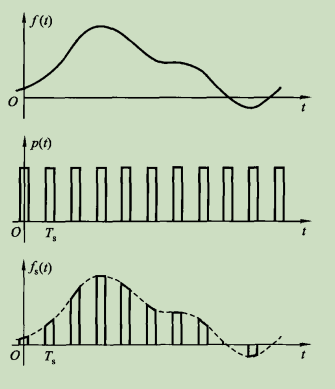对于我们而言，我们经常把 称为抽样函数，与这里所指的“抽样”或者“抽样信号”具有完全不同的含义。此外，这里的抽样也称为“采样”或“取样”。

抽样用来做什么？

我们通常对一个连续的信号进行抽样，使其变为抽样信号，然后再经量化、编码变成数字信号。这种数字信号经传输，然后进行上述过程的逆变换就可以恢复出原连续信号。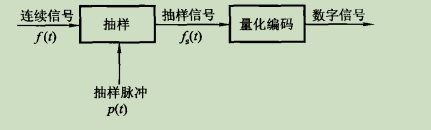就是说我们对模拟信号进行采样，就可以变成抽样信号，对抽样信号进行量化，就可以变成数字信号。

抽样信号的作用

假设一个模拟信号如下图所示：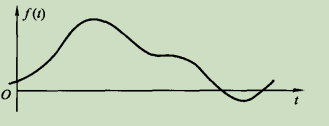我们知道自然界的信号都是模拟信号，但计算机不能直接处理模拟信号，计算机只能处理数字信号，并且只能处理有限长度的数据。因此，我们需要将模拟信号转换成数字信号，这就需要对其进行采样。

一般来说，采样越多，则与原信号与接近，但是我们要在尽可能获取少的值得同时又使其能恢复到原信号，这里面就牵扯到失真的问题。对于在不同的采样率下，信号的幅值是不同的，采样率越高，信号幅值失真越小。

展开全文• 1 典型函数傅里叶级数、傅里叶变换 1.1 单位冲激函数 单位冲激函数记作δ(t)\delta(t)δ(t)。定义为： {∫−∞∞δ(t)dt=1δ(t)=0(t≠0)(1.1) \left\{\begin{array}{l} {\int_{-\infty}^{\infty} \delta(t) \...

## 1 典型函数的傅里叶级数、傅里叶变换

### 1.1 单位冲激函数

单位冲激函数记作 δ ( t ) \delta(t) 。定义为：

{ ∫ − ∞ ∞ δ ( t ) d t = 1 δ ( t ) = 0 ( t ≠ 0 ) (1.1) \left\{\begin{array}{l} {\int_{-\infty}^{\infty} \delta(t) \mathrm{d} t=1} \\ {\delta(t)=0} \end{array} \quad(t \neq 0)\right. \tag{1.1}

如果冲激出现在 t = t 0 t=t_0 ,则定义为：

{ ∫ − ∞ ∞ δ ( t − t 0 ) d t = 1 δ ( t − t 0 ) = 0 ( t ≠ t 0 ) (1.2) \left\{\begin{array}{l} {\int_{-\infty}^{\infty} \delta\left(t-t_{0}\right) \mathrm{d} t=1} \\ {\delta\left(t-t_{0}\right)=0} \end{array} \quad\left(t \neq t_{0}\right)\right. \tag{1.2}

单位冲激函数的抽样特性（或称之为 筛选特性）：

∫ − ∞ ∞ δ ( t − t 0 ) f ( t ) d t = ∫ − ∞ ∞ δ ( t − t 0 ) f ( t 0 ) d t = f ( t 0 ) (1.3) \int_{-\infty}^{\infty} \delta\left(t-t_{0}\right) f(t) \mathrm{d} t=\int_{-\infty}^{\infty} \delta\left(t-t_{0}\right) f\left(t_{0}\right) \mathrm{d} t=f\left(t_{0}\right) \tag{1.3}

### 1.2 周期冲激函数序列的傅里叶级数

用符号 δ T ( t ) \delta_{T}(t) 来表示周期单位冲激序列，即

δ T ( t ) = ∑ n = − ∞ ∞ δ ( t − n T s ) (1.4) \delta_{T}(t)=\sum_{n=-\infty}^{\infty} \delta\left(t-n T_{s}\right) \tag{1.4}

展开成傅里叶级数

δ T ( t ) = ∑ n = − ∞ ∞ δ ( t − n T s ) = ∑ n = − ∞ ∞ F n e j n ω s t (1.5) \delta_{T}(t)=\sum_{n=-\infty}^{\infty} \delta\left(t-n T_{s}\right)=\sum_{n=-\infty}^{\infty} F_{n} e^{j n \omega_s t} \tag{1.5}

其中

F n = 1 T s ∫ − T s 2 T s 2 δ T ( t ) e − j n ω s t d t = 1 T s ∫ − T s 2 T s 2 δ ( t ) e − j n ω s t d t = 1 T s (1.6) \begin{aligned} F_{n} &=\frac{1}{T_{s}} \int_{-\frac{T_{s}}{2}}^{\frac{T_{s}}{2}} \delta_{T}(t) \mathrm{e}^{-jn \omega_{s} t} \mathrm{d} t \\ &=\frac{1}{T_{s}} \int_{-\frac{T_{s}}{2}}^{\frac{T_{s}}{2}} \delta(t) \mathrm{e}^{-j n \omega_{s} t} \mathrm{d} t \\ &=\frac{1}{T_{s}} \end{aligned} \tag{1.6}

那么

δ T ( t ) = 1 T s ∑ n = − ∞ ∞ e j n ω s t (1.7) \delta_{T}(t)=\frac{1}{T_{s}} \sum_{n=-\infty}^{\infty} e^{j n \omega_s t} \tag{1.7}

δ T ( t ) \delta_{T}(t) 的傅里叶变换为

F [ f ( t ) ] = 2 π ∑ n = − ∞ ∞ F n δ ( ω − n ω s ) (1.8) \mathscr{F}[f(t)]=2 \pi \sum_{n=-\infty}^{\infty} F_{n} \delta\left(\omega-n \omega_{s}\right) \tag{1.8}

F n = 1 T 1 F_n=\frac{1}{T_1} 代入式 1.8 {1.8} ，得

F ( ω ) = F [ δ T ( t ) ] = ω s ∑ n = − ∞ ∞ δ ( ω − n ω s ) (1.9) F(\omega)=\mathscr{F}\left[\delta_{T}(t)\right]=\omega_{s} \sum_{n=-\infty}^{\infty} \delta\left(\omega-n \omega_{s}\right) \tag{1.9}

1.9 {1.9} 式可见，周期单位冲激序列的傅里叶变换中，只包含位于 n ω s n\omega_s 处的冲激函数，强度是相等的，均等于 ω s \omega_s

## 2 抽样信号的傅里叶变换

主要看时域抽样。

令：

连续信号 f ( t ) f(t) 的傅里叶变换为

F ( ω ) = F [ f ( t ) ] (2.1) F(\omega)=\mathscr{F}[f(t)] \tag{2.1}

抽样脉冲序列 p ( t ) p(t) 的傅里叶变换为

P ( ω ) = F [ p ( t ) ] (2.2) P(\omega)=\mathscr{F}[p(t)] \tag{2.2}

抽样后信号 f s ( t ) f_s(t) 的傅里叶变换为

F s ( ω ) = F [ f s ( t ) ] (2.3) F_s(\omega)=\mathscr{F}[f_s(t)] \tag{2.3}

采用均匀抽样，抽样周期为 T s T_s ，抽样频率为 ω s = 2 π T s \omega_s=\frac{2\pi}{T_s}

一般情况下，抽样过程是抽样脉冲序列 p ( t ) p(t) 和和连续信号 f ( t ) f(t) 相乘，即：

f s ( t ) = f ( t ) p ( t ) (2.4) f_s(t)=f(t)p(t) \tag{2.4}

因为 p ( t ) p(t) 是周期信号，那么 p ( t ) p(t) 的傅里叶变换等于

P ( ω ) = 2 π ∑ n = − ∞ ∞ P n δ ( ω − n ω s ) (2.5) P(\omega)=2 \pi \sum_{n=-\infty}^{\infty} P_{n} \delta\left(\omega-n \omega_{s}\right) \tag{2.5}

其中

P n = 1 T s ∫ − T s 2 T s 2 p ( t ) e − j n ω s t d t (2.6) P_{n}=\frac{1}{T_{s}} \int_{-\frac{T_{s}}{2}}^{\frac{T_{s}}{2}} p(t) \mathrm{e}^{-\mathrm{j} n \omega_{s} t} \mathrm{d} t \tag{2.6}

根据频域卷积定理 F [ f 1 ( t ) ⋅ f 2 ( t ) ] = 1 2 π F 1 ( ω ) ∗ F 2 ( ω ) \mathscr{F}\left[f_{1}(t) \cdot f_{2}(t)\right]=\frac{1}{2 \pi} F_{1}(\omega) * F_{2}(\omega) ,可知

F s ( ω ) = 1 2 π F ( ω ) ∗ P ( ω ) = ∑ n = − ∞ ∞ P n F ( ω − n ω s ) (2.7) F_{s}(\omega)=\frac{1}{2 \pi} F(\omega) * \mathrm{P}(\omega)=\sum_{n=-\infty}^{\infty} P_{n} F\left(\omega-n \omega_{s}\right) \tag{2.7}

如果抽样脉冲序列 p ( t ) p(t) 为冲激序列，称之为“冲激抽样”或者“理想抽样”。那么：

p ( t ) = δ T ( t ) = ∑ n = − ∞ ∞ δ ( t − n T s ) (2.8) p(t)=\delta_{T}(t)=\sum_{n=-\infty}^{\infty} \delta\left(t-n T_{s}\right) \tag{2.8}

f s ( t ) = f ( t ) p ( t ) = f ( t ) ∑ n = − ∞ ∞ δ ( t − n T s ) (2.9) f_{s}(t)=f(t) p(t)=f(t) \sum_{n=-\infty}^{\infty} \delta\left(t-n T_{s}\right) \tag{2.9}

由上面的分析可得冲激抽样信号的频谱为：

F s ( ω ) = 1 T s ∑ n = − ∞ ∞ F ( ω − n ω s ) (2.10) F_{s}(\omega)=\frac{1}{T_{s}} \sum_{n=-\infty}^{\infty} F\left(\omega-n \omega_{s}\right) \tag{2.10}

2.10 {2.10} 式可以看出，经过冲激序列抽样后的信号傅里叶变换为原信号的傅里叶变换以 ω s \omega_s 为周期重复，幅度被 1 T s \frac{1}{T_s} 加权。

在实际中，可以近似认为是冲激抽样。

展开全文数字信号处理
• 在MATLAB中想要画一个sin函数是很容易的。...应该借鉴数字信号处理里的抽样思想：想生成函数x(t) = a*sin(2*pi*f0*t+ψ)，就应该考虑到抽样定理：以等时间间隔进行抽样，即x[n] = x[n*Ts] 其中Ts = ...

在MATLAB中想要画一个sin函数是很容易的。

比如:首先定义t = 0:0.01:10,然后画出y = sin(2*pi*t)就可以了，最多再加相角啊之类的参数。

但如果在C语言中应该如何自己制造一个sin函数呢？

应该借鉴数字信号处理里的抽样思想：

想生成函数x(t) = a*sin(2*pi*f0*t+ψ)，就应该考虑到抽样定理：以等时间间隔进行抽样，即x[n] = x[n*Ts] 其中Ts = 1/fs(fs是抽样频率)。

这时候想生成的函数x(t)就变成了x(t) = x(n*Ts) = x(n) = a*sin(2*pi*f0*n/fs+ψ) = a*sin(w*n+ψ)，其中w是数字角频率，这就将模拟时间信号转化成了数字信号。

举个例子，想生成函数y = sin(20*pi*t),用matlab直接生产的图形如下所示(取t=0:0.001：1)

matlab程序为

t = 0:0.001:1;

y = sin(20*pi*t);

plot(t,y);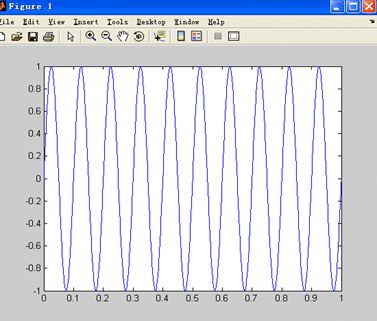如果想用C语言来实现怎么办呢？

从原函数y = sin(20*pi*t)可知：

f0 = 10,设fs = 40，n = 0:10(这里的n都是整数)来看看，此时x(n) = sin(2*pi*f0*n/fs)，matlab程序为

clear all;

f0=10;

fs=40;

N=10;

n=0:N-1;

y=sin(2*pi*n*f0/fs);

画出来的图形如下图：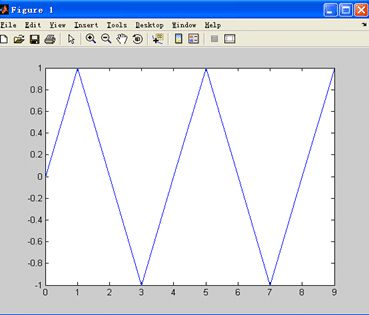现在函数式为x[n] = sin(n*pi/2),周期是4

为什么看着不像以前的正弦函数了？因为这是对原函数抽样后得到的，而且它的横坐标已经变成了n而不是t。为了让抽样后的x[n]看起来更像x[t]那么方法只有一个，就是增加抽样率fs。当抽样率为80时x[n] = sin(n*pi/4),

f0=10;

fs=80;

N=10;

n=0:N-1;

plot(n,y);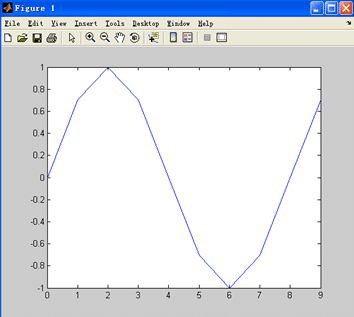看着更像x(t)了！这是因为抽样点更密了。

所以产生x(t)=a*sin(2*pi*f0*t+ψ)的正弦波步骤：

对x(t)进行以fs为抽样频率的抽样后得到

x(n)=a*sin(2*pi*f0*n/fs+ψ)=a*sin(w*n+ψ)

画的时候指明a，f0，fs，ψ就可以对任意sin函数进行画图

那么，如何知道现在画出来的x(n)就是以前的x(t)呢？以前是T = 0.1S,现在变成了T = 8，在这种情况下如何换算呢？就有FFT了！

下一篇文章我们再继续。

展开全文• 离散时间傅里叶变换Matlab实现发布时间：2018-09-22 17:24,浏览次数：1077, 标签：Matlab一、代码实现%%离散时间傅里叶变换DTFT%若x(t)=cos(2*pi*t)，取样时间为0.1s,得到一个32的有限序列，利用matlab计算他的DFT并...
• 第3章 傅里叶变换 1.傅里叶级数定义及适用条件 2.常见周期信号的频谱,非周期性信号的频谱 3.傅里叶变换的定义及适用条件及性质 4.周期信号的傅里叶变换 5.抽样定理 6.功率频谱与能量频谱 7.系统频域分析法 8....
• 本节为opencv数字图像处理（4）：频率域滤波的第二小节，取样和取样函数傅里叶变换，主要包括：取样、取样函数傅立叶变换、取样定理以及根据取样数据重建原函数的数学推导。opencv 数字图像处理
• 在第9部分学习笔记中，我们导出了分布傅里叶变换，用以解决普通傅里叶变换难以解决的一些问题。本文的内容就是在此基础上进一步进行讨论。 首先是分布傅里叶变换的导数相关性质 ⟨T′,φ⟩=∫∞−∞T′(x)φ(x...
• 线性系统和傅里叶变换研究的核心是冲激及其取样特性。连续变量t在t= 0处的单位冲激表示： 满足等式        物理上，如果我们把t解释为时间，那么一个冲激可看成是幅度无限、持续时间为0....卷积
• 龙源期刊网 http://www.doczj.com/doc/dafc8a4a82d049649b6648d7c1c708a1294a0aed.html用MATLAB制作二元傅里叶变换计算全息图作者：王永瑛张光明姜先策吴娜娜来源：《商情》2010年第18期[摘要]计算全息技术作为现代...
• 作一维傅里叶变换： (1)F(u)=∫−∞∞f(x)e−j2πuxdx=∫−∞∞e−j2πuxdx=δ(u)F(u)=\int_{-\infty}^{\infty}f(x)e^{-j2\pi ux}dx=\int_{-\infty}^{\infty}e^{-j2\pi ux}dx=\delta(u)\tag1F(u)=∫−∞∞​f(x)e−j...
• 数字信号处理实验八 离散傅里叶变换 一、实验目的 1．通过实验加深对DFT 的理解。... 当信号在时域和频域中都是抽样的离散函数时，按照傅里叶变换的概念，他们在两个域中也必然是周期的。............matlab 数字信号处理
• ## sin2t的傅里叶变换

万次阅读 2021-01-12 23:16:16
常用函数傅里叶变换(典型非周期信号的频谱) 1、 门函数 F(w)= 2 sin w? ...表6.3 f (t ) = 1 2π +∞ ?∞ 常用的连续傅里叶变换对及其对偶关系 dω F (ω ) = +∞ ?∞ ∫ F (ω )e jωt ∫ f (t )e ? jωt .........
• 摘要：傅里叶变换的核心在于，“任何连续周期信号可以由一组适当的正弦曲线组合而成”，在这个基础上对信号的中特定频率的正弦波进行分解或者重组，基于频率方面分析波形。1、傅里叶变换的意义近似周期...算法 python 机器学习 人工智能
• 采用坐标扩展变换，突破了快速傅里叶变换计算过程中输入屏和衍射屏空间尺度必须相同、抽样格点必须等间隔的限制，使上述问题得到解决，可以得到更加详细的聚焦光束光场分布。同时，采用分两步计算的思想，避免了计算...
• 本文是对知乎上大佬的学习， 其知乎名为逸珺：傅里叶变换、拉普拉斯变换、Z 变换的...数学变换：指函数从原向量空间变换为另一个向量空间，或从集合x到另一个集合Y的可逆函数。 如旋转变换，平移，对称等等 数学中..
• 0.1. 定义 0.1.1. 连续 0.1.2. 离散 0.2. 性质 0.2.1. 分离性 0.2.2. 位移定理 0.2.3. 周期性 0.2.4. 共轭对称性 0.2.5. 旋转性 0.2.6. 加法定理 0.2.7. 平均值 ...0.3. 快速傅里叶变换 0.3...图像处理 数学
• 数字图像处理(Digital Image Processing)是通过计算机对图像进行去除噪声、增强、复原、分割、提取...傅里叶变换（一种正交变换） 一维傅里叶变换对 二维傅里叶变换对 二维离散傅里叶变换的性质 空间域抽样间...数字图像处理 计算机视觉 学习笔记 人工智能
• 第一个重要的点是周期性抽样串及其傅里叶变换，我们之所以要提及周期性抽样串，是因为它是连续信号到离散信号的桥梁，而且由于其傅里叶变换也是一个频域的周期抽样串所以可以用来对信号进行离散和周期化。...算法
• 实验一 序列的傅里叶变换和离散傅里叶变换及其关系 一、[实验目的] 1.掌握序列傅里叶变换 DTFT 的计算机实现方法； 2.掌握序列 DFT 基本理论及其计算机实现方法； 3.掌握序列傅里叶变换 DTFT 和序列 DFT 的关系； 4 ...matlab 算法
• 傅里叶变换是一种函数在空间域和频率域的变化，从空间域到频率域的变换傅里叶变换，而从频率域到空间域的变换傅里叶的反变化。 频域：是指在对函数或信号进行分析时，分析其和频率有关 部分，而不是和时间有关...深度学习
• 函数傅里叶级数不含正弦项，F(nw1)为实函数 偶函数傅里叶级数不含余弦项，F(nw1)为虚函数 周期信号傅里叶变换 非周期信号傅里叶变换 注： 理论上只有满足绝对可和条件的非周期信号才能傅里叶变换，而周期信号...
• DFT:(Discrete Fourier Transform)离散傅里叶变换傅里叶变换在时域和频域上都呈离散的形式，将信号的时域采样变换为其DTFT的频域采样。在形式上，变换两端（时域和频域上）的序列是有限长的，而实际上这两组序列...
• 东北电力大学信号与系统课程专题报告-基于傅里叶变换的电力系统谐波分析，电气工程及其自动化专业。
• 从前面我们已经知道，非周期连续函数傅里叶变换如下 F(ω)=∫−∞+∞f(t)e−iωtdt F(\omega)=\int ^{+\infty}_{-\infty}f(t)e^{-i\omega t}dt F(ω)=∫−∞+∞​f(t)e−iωtdt 单位冲激函数的傅里叶变换如下 ...算法 卷积 傅立叶分析
• f ( t ) ← → F ( j ω ) f(t) \leftarrow \rightarrow F(j \omega) f(t)←→F(jω) 矩形脉冲取样： s ( t ) s(t) s(t)是周期为 T s T_s Ts​的矩形脉冲信号 (或称为开关函数) 周期信号的频谱是脉冲序列。...算法
• 傅里叶1768年生于法国,1807年提出“任何周期信号都可用正弦函数级数表示”, 1822年在“热的分析理论”一书中再次提出。1829年狄里赫利给出傅里叶变换收敛条件。傅里叶变换得到大规模的应用，...
• 基于MATLAB去更形象直观地其学习理解傅里叶级数和傅里叶变换，观察频谱的变化，相位的变换，通过例子来明白傅里叶变换与FFT的区别，以及掌握常用的信号处理MATLAB函数
• ## 傅里叶变换算法

千次阅读 2022-05-04 08:40:55
傅里叶变换的核心在于，“任何连续周期信号可以由一组适当的正弦曲线组合而成”，在这个基础上对信号的中特定频率的正弦波进行分解或者重组，基于频率方面分析波形。 一、傅里叶变换的意义    近似周期性的方...算法 c语言...